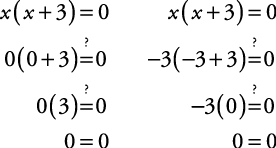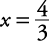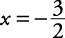## Solving Equations by Factoring

Factoring is a method that can be used to solve equations of a degree higher than 1. This method uses the zero product rule.

If ( a)( b) = 0, then

Either ( a) = 0, ( b) = 0, or both.

##### Example 1

Solve x( x + 3) = 0.

x( x + 3) = 0

Apply the zero product rule.Check the solution.The solution is x = 0 or x = –3.

##### Example 2

Solve x 2 – 5 x + 6 = 0.

x 2 – 5 x + 6 = 0

Factor.

( x – 2)( x – 3) = 0

Apply the zero product rule.The check is left to you. The solution is x = 2 or x = 3.

##### Example 3

Solve 3 x(2 x – 5) = –4(4 x – 3).

3 x(2 x – 5) = –4(4 x – 3)

Distribute.

6 x 2 – 15 x = –16 x + 12

Get all terms on one side, leaving zero on the other, in order to apply the zero product rule.

6 x 2 + x – 12 = 0

Factor.

(3 x – 4)(2 x + 3) = 0

Apply the zero product rule.The check is left to you. The solution isor.

##### Example 4

Solve 2 y 3 = 162 y.

2 y 3 = 162 y

Get all terms on one side of the equation.

2 y 3 – 162 y = 0

Factor (GCF).

2 y( y 2 – 81) = 0

Continue to factor (difference of squares).

2 y( y + 9)( y – 9) = 0

Apply the zero product rule.The check is left to you. The solution is y = 0 or y = –9 or y = 9.